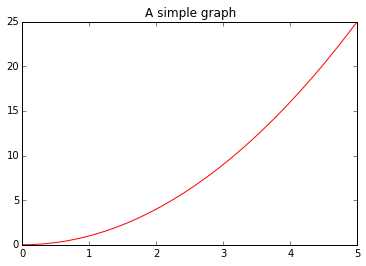CoCalc Public Filessupport / 2014-11-05-Nature.html
Description: IPython html version of support/2014-11-05-Nature.ipynb
Compute Environment: Ubuntu 18.04 (Deprecated)
2014-11-05-Nature[Rackspace graphic]

Welcome! You have just launched a live example of an IPython Notebook, an interactive computational environment that lets you combine raw code, text, mathematics, plots and rich media into one document. You can edit anything in this temporary demonstration notebook - including the text you are reading. (A real notebook could be saved and shared with colleagues). It will showcase some of the IPython notebook's capabilities for researchers. You can find more examples at this gallery of interesting IPython Notebooks.

The box below (known as a 'cell') contains code to plot the graph of $y=x^2$. [The blue comments marked by # explain what the code does]. Double-click on the cell to enter it. Now press SHIFT+ENTER, or press the play key () in the top menubar, to run its code. A full tutorial for using the notebook interface is available here.

In :
# imports a 2D/3D graphics plotting library called matplotlib
# which can generate scientific figures, and instructs the notebook
import matplotlib.pyplot as plt
%matplotlib inline

# creates an array of 30 values for x equally spaced from 0 to 5.
import numpy as np
from numpy import *

x = np.linspace(0, 5, 30)
y = x **2

plt.plot(x, y, 'r')
plt.title('A simple graph');Above, you should see a chart of $y=x^2$.

You can tweak this code, and re-execute it within the notebook. For example, replace "y = x ** 2" with "y = sin(x)". (For a list of valid commands, see the numpy reference manual). Be sure to update the descriptive text that support rich math using $\LaTeX$ notation.

IPython notebooks can run interactive plots. Aron Ahmadia (ERDC-CHL) and David Ketcheson (KAUST) created the following example to illustrate the perils of aliasing: when a rapidly-changing periodic signal is sampled too infrequently, creating a faulty impression.

Ketcheson explains:

As an undergraduate, I did some observational astronomy looking at variable stars. These are stars whose brightness oscillates, usually on a fairly regular basis. Many published results claim to measure how quickly the star's brightness oscillates - but actually report the oscillations at some multiple of the real answer, due to insufficient observation and (as a result) aliasing

This notebook shows how trying to reconstruct a simple sine wave signal from discrete measurements can fail. The sliders allow you to adjust the frequency of the underlying periodic sine wave signal (represented by the wavenumber, $p$), and also how often the signal is sampled (represented by the number of discrete grid points, $m$, at which the amplitude of the underlying signal is measured). Get it wrong, and a high-frequency sine wave is measured as a lower-frequency signal.

In []:
%matplotlib inline
import numpy as np
import matplotlib.pyplot as plt

from IPython.display import display, HTML
from IPython.html import widgets
from IPython.html.widgets import interact, interactive

def plot_sine(wavenumber=4.0,grid_points=12,plot_sine='On'):
"Plot sin(2*pi*p), sampled at m equispaced points."
x  = np.linspace(0,1,grid_points+2); # grid
xf = np.linspace(0,1,1000) # fine grid
y  = np.sin(wavenumber*np.pi*x)
yf = np.sin(wavenumber*np.pi*xf)
fig = plt.figure(figsize = (8, 6));
if plot_sine == 'On':
ax.plot(xf, yf, 'r-', linewidth=2);
ax.plot(x,  y,  'o-', lw=2)

interact(plot_sine, wavenumber=(1.0,44.0), grid_points=(10, 16, 1), plot_sine=(('On','Off')));


Run previous cell, then set the gridpoint with above slider to 13 and the wavenumber w to be : $22 \leq w \leq 24$ , see how the measured signal has a lower frequency than the real one.

Since it runs any code, an IPython notebook can be used to import libraries that permit commands for more complicated algorithms (including anything that runs in the computer languages R, Python, Julia, and others). Scikit-image, for example, is a collection of algorithms that can be used for image processing.

In []:
%matplotlib inline
from IPython.html.widgets import interact, interactive, fixed
from IPython.display import display
import matplotlib.pyplot as plt
import skimage
from skimage import data, filter, io
i = data.coffee()
io.Image(i)
lims = (0.0,1.0,0.01)

@interact
def edit_image(image: fixed(i), σ:(0.0,30.0,0.1)=0.1, r:lims=1.0, g:lims=1.0, b:lims=1.0):
new_image = filter.gaussian_filter(image, sigma=σ, multichannel=True)
new_image[:,:,0] = r*new_image[:,:,0]
new_image[:,:,1] = g*new_image[:,:,1]
new_image[:,:,2] = b*new_image[:,:,2]
new_image = io.Image(new_image)
plt.imshow(new_image)
return new_image

In []: